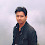### LoRa: Symbol Generation

LoRa is a chirp spread spectrum modulation based wireless networking standard.

Chirp:
Chirp stands for 'Compressed High Intensity Radar Pulse'. It is a signal which frequency either increase or decrease with time. It is very common is sonar and radar. It is also used in spread spectrum.

Chirp Spread Spectrum was developed for radar applications. Chirp signals have constant amplitude and pass the whole bandwidth in a linear or non-linear way from one end to another end in a certain time. Chirp spread spectrum uses complete bandwidth to transmit signals. If the frequency changes from lowest to highest, it is call up-chirp and if the frequency changes from highest to lowest, we call it down-chirp. Following is the example of linear up-chirp:

- CSS (chirp spread spectrum) techniques helps to transmit signals for very large distances.
- Bandwidth, time product is always greater than one (B*T > 1).
- Chirp spread spectrum is resistive to Doppler shift.
- It is used for low power and low data rates.

LoRa uses three different bandwidth: 125kHz, 250kHz and 500kHz (125kHz is used here). LoRa symbols are modulated over a up-chirp of 125kHz bandwidth and different orthogonal (almost) spreading factors are used based on data rate requirement and channel conditions. LoRa uses SF7 to SF12 spreading factors.
Here is a spectrogram or different Spreading Factors:
(For matlab codes of above plot please refer to the post )

LoRa physical layer includes 8 preamble symbols, 2 synchronisation symbols, physical payload and optional CRC.
- SF8 takes exact twice the time of SF7 and SF9 takes exact twice time of SF8.
- Symbol Rate(Rs), Bandwidth(BW) and Spreading Factor(SF) relation:
Rs = BW / (2^SF)
- Higher the Spreading Factor -> Higher the over-the-air time.
- Lower the Spreading Factor -> Higher the Data Rate.

Here is spectrogram of LoRa physical layer:

First 8 up-chirp symbols are preamble symbols used to detect LoRa chirps, next 2 down-chirp symbols are synchronisation symbols used for timing synchronisation followed by the 5 modulated symbols (payload). The jump in the frequency represents the modulated symbol.

References:

If you are a research student and want to sell your work on my Blog here, please reach me on sakshama.ghosliya@gmail.com

1.How many beats are there in a symbol?

1.Number of bits in a symbol depends on Spreading Factor used. This is how you encode your bits on a chirp. In short 'Number of bits in a symbol' = 'SF used'.

2.Hi

If SF8 takes twice the time of SF7, but I also have twice the amount of bits per symbol (SF7=128 bits, SF8=256 bits) is the bitrate then not equal independent of the SF?

Thanks

3.Hello Friend,

SF7 means you can transmit 7 bits (0 to 127). SF8 means 8 bits can be transmitted.
I hope you have gone through this: http://www.sghoslya.com/p/lora_9.html

4.Hello Friend,

This is what i understand. But what I don't understand is the following:
If one symbole(Chirp) takes Ts time. Ts = (2^SF)/BW
Ts=(2^7)/125k=1,024 mili second
Ts=(2^8)/125k=2,024 mili second
So one symbol takes twice the time with SF8 then SF7.
With SF8 I can send one symbol that will contain 256 bits of data.
In the same symbol period of SF8, I can send two symbols with SF7, but only with 128 bits of data per symbol, what totals to 256 bits.
So in this explanation the data rate will be independent of the spread factor. I know that this not true, because of the following: Rb=SF*(1/((2^SF)/BW))
Can you explain what I'm doing wrong here?

Anyway thank for this amazing LoRa tutorial, I learned a lot from it.

5.Hello Friend,

Read the text clearly. SF8 means 8 bits per symbol not 256 bits per symbol. And 8 bits means 0-255 decimal number, 00000000 to 11111111 binary number. Hope you understood this time.

2.Hi,

can you post matlab code of last spectrogram, entitled as "LoRa symbols [8 preamble, 2 Sync, 5 Symbols]". I want to see how and why LoRa makes frequency shifts after preamble and sync word.

1.Hello Umber,

You can check codes under "LoRa Decoding" page (http://sakshamaghoslya.blogspot.in/p/matlab-codes-for-lora-decoding.html) of this blog. If you will change the number of symbols and the symbols, you will get this graph.

3.what is the relation between Spreading factor,Bandwidth and CodingRate in LoRa?

1.4.Dear Sakshama Ghoslya,

I want to send 07,56,45, A3 hexa data with CSS (00000111,01010110,01000101,10100011). Spreading factor (SF) is 8. So how many chirp symbols do I use? four? The information I want to be sure is shudder. The useful serial I will send is the encoded data, I use it According to the spreading factor (eg SF = 8) should I consider 8 bit symbols?
It's the information I want to make sure. I want to send a serial encoded binary data via CSS modulation. I'll use the Spreading factor 8. In this case, I must generate symbols with 8 bits using the serial encoded data. These symbols are now Chirp symbols. Is this the right way of thinking?

1.hello,

5.Hi, can i use the second and third pictures for my Thesis project? of course i will mention your site as source. Let me know, great work anyway!

1.Hi Leonardo, Yes you can use. Best of luck for your thesis.

6.Hi!
I was wondering if I could use your sources here in my thesis paper?

1.Hi, Many people have used it as a reference.

7.1.8.